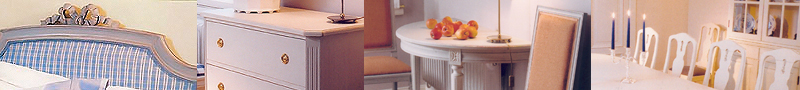S T O L A R  |   K A R M S T O L A R  |   T A B U R E T T E R   &   B Ä N K A R  |   G R I P S H O L M S M Ö B L E RS O V R U M S M Ö B L E R  |   S P E G L A R  |   B Y R Å E R  |   B O R D  |   S K R I V B O R DV I T R I N S K Å P   &   B O K H Y L L O R  |   S K Ä N K A R  |   V Ä G G S K Å P   &   T A L L R I K S H Y L L O R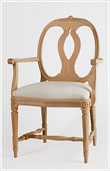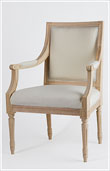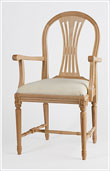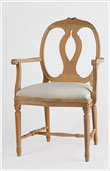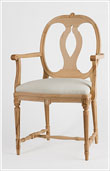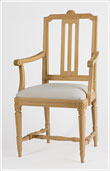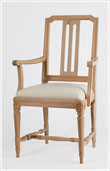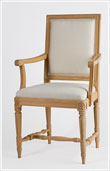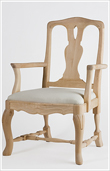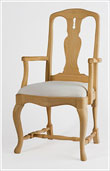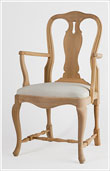© S O LARSSON STILMÖBLER EFTR. AB  |  E-POST: LARSSON.STILMOBLER@TELIA.COM  |  PRODUKTION: BÅS